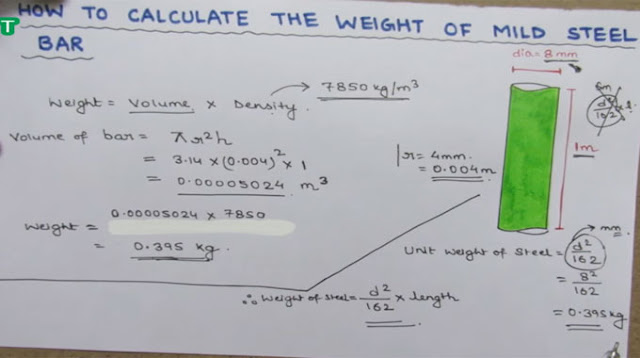Tutorial on calculating the weight of steel

# Tutorial on calculating the weight of steelIn the following tutorial video, one can understand and learn the process of calculating the weight of steel. There are several formulas and methods that we can use. But the most famous and easiest methods are “density method” and the D square 162 method.
Watch the following video tutorial to get more detail information.

Continue Reading about the "Tutorial on calculating the weight of steel" on the next page below